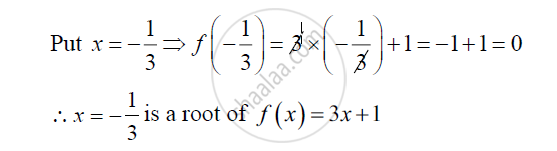Share

Books Shortlist

# Verify Whether the Indicated Numbers is Zeroes of the Polynomials Corresponding to Them in the Following Case: F ( X ) = 3 X + 1 , X = − 1 3 - CBSE Class 9 - Mathematics

ConceptZeroes of a Polynomial

#### Question

Verify whether the indicated numbers is zeroes of the polynomials corresponding to them in the following case:

f ( x ) = 3x +1, x = - 1/3

#### Solution

f ( x ) = 3x +1, x = - 1/3

We have

f ( x) = 3x +1

Putx=-1/3⇒f(-1/3)=3xx(-1/3)+1=-1+1=0

∴x=-1/3 is root of f(x)=3x+1

Is there an error in this question or solution?

#### APPEARS IN

RD Sharma Solution for Mathematics for Class 9 by R D Sharma (2018-19 Session) (2018 to Current)
Chapter 6: Factorisation of Polynomials
Ex.6.20 | Q: 2.1 | Page no. 8
Solution Verify Whether the Indicated Numbers is Zeroes of the Polynomials Corresponding to Them in the Following Case: F ( X ) = 3 X + 1 , X = − 1 3 Concept: Zeroes of a Polynomial.
S